×
Get Full Access to Organic Chemistry - 7 Edition - Chapter 2 - Problem 48p
Get Full Access to Organic Chemistry - 7 Edition - Chapter 2 - Problem 48p

×

# Which is a stronger base?a. HS? or HO? c. CH3OH or CH3O?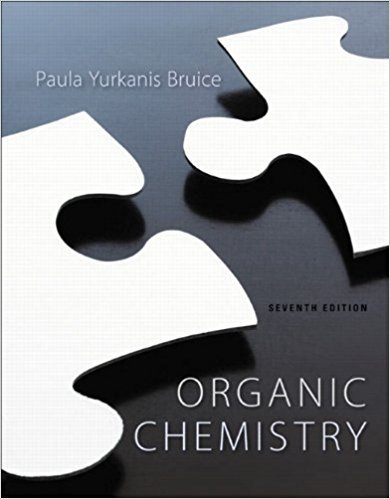ISBN: 9781269406772 80

## Solution for problem 48P Chapter 2

Organic Chemistry | 7th Edition

• Textbook Solutions
• 2901 Step-by-step solutions solved by professors and subject experts
• Get 24/7 help from StudySoup virtual teaching assistantsOrganic Chemistry | 7th Edition

4 5 1 265 Reviews
25
3
Problem 48P

Which is a stronger base?

a. $$H S^{-} \text {or } H O^{-}$$                c. $$\mathrm{CH}_{3} \mathrm{OH} \text { or } \mathrm{CH}_{3} \mathrm{O}^{-}$$                e. $$\mathrm{CH}_{3} \mathrm{COO}^{-} \text {or } \mathrm{CF}_{3} \mathrm{COO}^{-}$$

b. $$\mathrm{CH}_{3} \mathrm{O}^{-} \text {or } \mathrm{CH}_{3} \overline{\mathrm{N}} H$$                d. $$\mathrm{Cl}^{-} \text {or } \mathrm{Br}^{-}$$                        f. $$\mathrm{CH}_{3} \mathrm{CHClCOO}^{-} \text {or } \mathrm{CH}_{3} \mathrm{CHBrCOO}^{-}$$

Equation Transcription:Text Transcription:

HS^- or HO^-

CH_3 O^- or CH_3 overline N H

CH_3 OH or CH_3 O^-

Cl^- or Br^-

CH_3 COO^- or CF_3 COO^-

CH_3 CHClCOO^- or CH_3 CHBrCOO^-

Step-by-Step Solution:

Step 1 of 6

Here we have to find out which is stronger base among the given molecules.

It is known that the relative acidity depends on the following two factors:

1.  Atomic size

2.  A Stronger base has weaker conjugate acid.

a.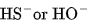Here the bases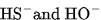are formed from its conjugate acid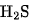and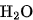. Hereis the stronger acid than that ofdue to the presence of S-atom, which has big atomic size than O-atom.

The conjugate base of the strong acid is less basic, thus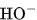is the stronger base than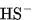.

__________________________

Step 2 of 6

Step 3 of 6

## Discover and learn what students are asking

Calculus: Early Transcendental Functions : Space Coordinates and Vectors in Space
?In Exercises 1-4, plot the points in the same three-dimensional coordinate system. (a) (5, -2, 2)

Calculus: Early Transcendental Functions : Surface Integrals
?In Exercises 1 - 4, evaluate $$\int_{S} \int(x-2 y+z) d S$$. $$S: z=2, x^{2}+y^{2} \leq 1$$

Statistics: Informed Decisions Using Data : Discrete Probability Distributions
?State the criteria that must be met for an experiment to be a binomial experiment.

Statistics: Informed Decisions Using Data : Tests for Independence and the Homogeneity of Proportions
?Prenatal Care An obstetrician wants to learn whether the amount of prenatal care and the wantedness of the pregnancy are associated. He randomly selec

Statistics: Informed Decisions Using Data : Comparing Three or More Means (One-Way Analysis of Variance)
?Corn Production The data in the table represent the number of corn plants in randomly sampled rows (a 17-foot by 5-inch strip) for various types of pl

Unlock Textbook Solution